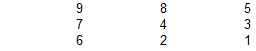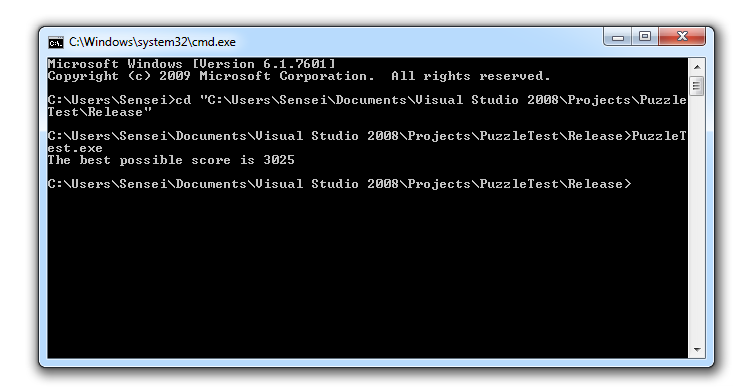## Recommended Posts

Your Mission is to find the best score for the pattern

a  b  c
d  e  f
g  h  i     where a to i stand for one of 1 2 3 4 5 6 7 8 9 uniquely !

for example    1  2  3
4  5  6
7  8  9

The Pattern Score is calculated as :

=  Rows–Row Products+Columns–Column Products–Product of Diagonals–Product of Corners–Product of Diamonds

Which means in the example pattern the Score is :
= 123–6+456–120+789–504+147–28+258–80+369–162–45–105–189–384
=519

Find the Pattern for getting the HIGHEST SCORE !

##### Share on other sites

Seems like it should be easy if you just set up the permutations in a spreadsheet and plug in the formula-- maybe I'll play with it later.

##### Share on other sites

In such puzzles I want to run Visual Studio Express C/C++ so it'll do it for me..Didn't have time for it, so the first attempt 3023.

SpoilerEdited by Sensei

##### Share on other sites

Hi Sensei,

I got 3025 and if you can better it it will be interesting !

Is there a Combination for a score more than 3025 ?

A more involved Puzzle will be :

Your Mission is to find the best score for the pattern

a  b  c
d  e  f
g  h  i     where a to i stand for one of 1 2 3 4 5 6 7 8 9 uniquely !

for example     9  7  5
8  4  3
6  2  1

The Pattern Score is calculated as :

=  Rows – Columns + Product of Diagonals – Product of Corners + Product of Ribs – Product of Diamond
+  Sum of Corners + Sum of Squares of Diamonds + Cube of the Center Number

Which means in the example pattern the Score is :
= 975+843+621 –986–742–531 + 9*4*1+6*4*5–9*5*1*6 +8*4*3+7*4*2– 8*7*3*2 +9+5+1+6+8^2+7^2+3^2+2^2+4^3
= 93

Find the Pattern for getting the HIGHEST SCORE !

##### Share on other sites
1 hour ago, Commander said:

I got 3025 and if you can better it it will be interesting !

Is there a Combination for a score more than 3025 ?

There are two mirror answers of 3025:

/*
* PuzzleTest v1.0 (c) 2019 created by Sensei.
*/
#include <stdio.h>

void decode( int index, int data[ 3 ][ 3 ] )
{
for( int i = 0; i < 9; i++ )
{
int value = index % 10;
data[ i / 3 ][ i % 3 ] = value;
index /= 10;
}
}

bool has_zeroes( const int data[ 3 ][ 3 ] )
{
for( int j = 0; j < 3; j++ )
{
for( int i = 0; i < 3; i++ )
{
if( data[ j ][ i ] <= 0 ) return( true );
}
}
return( false );
}

bool has_duplicates( const int data[ 3 ][ 3 ] )
{
bool result = false;
int digits[ 10 ] = { 0 };
for( int j = 0; j < 3; j++ )
{
for( int i = 0; i < 3; i++ )
{
int value = data[ j ][ i ];
if( digits[ value ] != false ) return( true );
digits[ value ] = true;
}
}
return( false );
}

int calc_score_column( const int data[ 3 ][ 3 ], int column )
{
return( data[ 0 ][ column ] * 100 + data[ 1 ][ column ] * 10 + data[ 2 ][ column ] );
}

int calc_score_column_product( const int data[ 3 ][ 3 ], int column )
{
return( data[ 0 ][ column ] * data[ 1 ][ column ] * data[ 2 ][ column ] );
}

int calc_score_row( const int data[ 3 ][ 3 ], int row )
{
return( data[ row ][ 0 ] * 100 + data[ row ][ 1 ] * 10 + data[ row ][ 2 ] );
}

int calc_score_row_product( const int data[ 3 ][ 3 ], int row )
{
return( data[ row ][ 0 ] * data[ row ][ 1 ] * data[ row ][ 2 ] );
}

int calc_score( const int data[ 3 ][ 3 ] )
{
int score = 0;
for( int i = 0; i < 3; i++ )
{
score += calc_score_column( data, i );
score -= calc_score_column_product( data, i );
score += calc_score_row( data, i );
score -= calc_score_row_product( data, i );
}
score -= data[ 0 ][ 0 ] * data[ 1 ][ 1 ] * data[ 2 ][ 2 ];
score -= data[ 0 ][ 2 ] * data[ 1 ][ 1 ] * data[ 2 ][ 0 ];
score -= data[ 0 ][ 1 ] * data[ 2 ][ 1 ] * data[ 1 ][ 0 ] * data[ 1 ][ 2 ];
score -= data[ 0 ][ 0 ] * data[ 0 ][ 2 ] * data[ 2 ][ 0 ] * data[ 2 ][ 2 ];
return( score );
}

int calc_score( int index )
{
int data[ 3 ][ 3 ] = { 0 };
decode( index, data );
if( has_zeroes( data ) ) return( -1 );
if( has_duplicates( data ) ) return( -1 );
return( calc_score( data ) );
}

void show( const int data[ 3 ][ 3 ] )
{
for( int j = 0; j < 3; j++ )
{
for( int i = 0; i < 3; i++ )
{
printf( "%d ", data[ j ][ i ] );
}
printf( "\n" );
}
printf( "\n" );
}

void show( int index )
{
int data[ 3 ][ 3 ] = { 0 };
decode( index, data );
show( data );
}

int main( int argc, int argv[] )
{
#if 0
int data[ 3 ][ 3 ] =
{
{ 1, 2, 3 },
{ 4, 5, 6 },
{ 7, 8, 9 },
};
show( data );
printf( "Score is %d", calc_score( data ) );
#endif
#if 0
int data[ 3 ][ 3 ] =
{
{ 9, 8, 5 },
{ 7, 4, 3 },
{ 6, 2, 1 },
};
show( data );
printf( "Score is %d", calc_score( data ) );
#endif
#if 1
// Brute-force scan of the all possible combinations.
// Some of them (containing zeroes) will be ignored inside of calc_score() function.
int max_score = 0;
for( int i = 0; i < 1e9; i++ )
{
int score = calc_score( i );
if( score > max_score )
{
max_score = score;
}
}
printf( "The best possible score is %d\n", max_score );
#endif
return( 0 );
}ps. I don't normally use plain ANSI C, rather C++, but it grew and grew while writing..

Edited by Sensei

##### Share on other sites

Yes,

These are the two combinations !

 9 8 4 984 976 288 378 165 7 5 3 753 852 105 80 216 6 2 1 621 431 12 12 336 2358 2259 405 470 717 3025 9 7 6 976 984 378 288 165 8 5 2 852 753 80 105 216 4 3 1 431 621 12 12 336 2259 2358 470 405 717 3025

You are very good in programming !

I have so far been able to use Excel for these Puzzles with success !

## Create an account

Register a new account